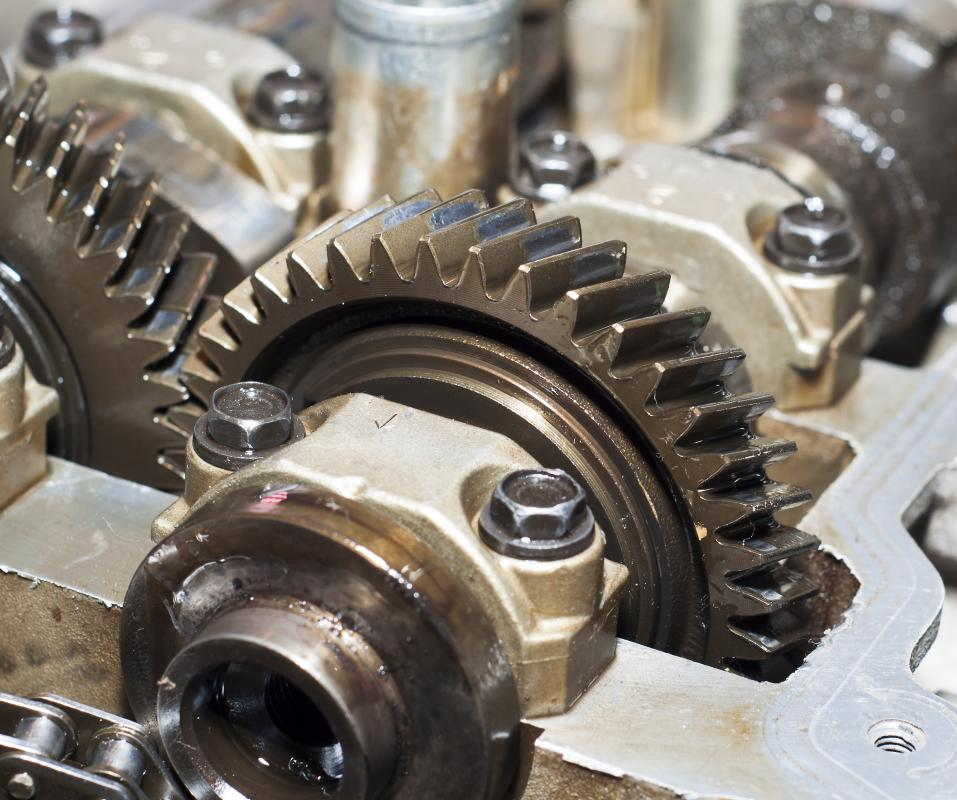# What is Gear Speed?

M. McGee
M. McGee

Gear speed is a measurement of how quickly a gear spins, often in relation to the rotations of other gears. The relative size of gears in a geared system is a very tightly controlled factor. This is because gears are able to transfer power from one system into another. The size of the gears directly influences the gear speed, which directly influences the amount of power moved from one area of the system to another. Gear speed is generally expressed in revolutions per minute (RPM) or as a ratio to another system.Gear speed is a measure of how quickly a gear turns.

The basics of gear rotation are actually quite simple. Since gears have interlocking teeth, they are forced to move at a constant speed. If one gear stops, so do all other connected gears. This, in turn, stops every gear they are connected to, which stops even more, and so on. The only alternative to stopping is a total mechanical failure; the gear’s teeth break off, or the gear itself comes apart.

To determine the gear speed of two interconnected gears, all a person needs to do is count their teeth. If the powered gear has 25 teeth and the moved gear has 50, then the gear ratio is 25:50 or 1:2. One revolution of the powered gear is equal to one half of a revolution of the driven gear. This means the powered gear moves twice as fast as the driven gear, and since the gears are irrevocably connected, it always will.

This ratio not only expresses gear speed, but power as well. Since the powered gear turns faster than the driven gear, it passes more power into the system. The energy the powered gear makes in two rotations equals the power the larger gear makes in one, so it has multiplied its available power by two. This is why lower gears in a car transfer more power to the wheels; the slower-moving low gears have more power than the faster moving high gears. The engine’s power is multiplied by the ratio of the gearing system.

The other common way of expressing gear speed is RPM. This is usually a pretty straightforward process that involves counting how many revolutions a gear makes in a minute or finding how long it takes to make one rotation and multiplying or dividing that number into a minute. If a gear makes 1.5 revolutions per second, then the user would simply multiply that to find it has an RPM of 90. If it makes one rotation every four minutes, then the user would divide to find its RPM of .25.

## You might also Like